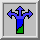# The Two-Dimensional Random Walk2-D Random Walk Java Applet

Suppose that the ant is not forced to step just along a line, but can move in four mutually perpendicular directions when walking away from the lamp post. This type of movement is called a {\it 2-dimensional random walk}.

For example, an ant is standing in the center of a 11 by 11 grid, as shown in the figure. Each grid square is the size of one step. The ant can move one step at a time in one of four directions: north, south, east, or west. The ant cannot move diagonally or take more than one step at a time. If the ant walks off the edge of the grid, it cannot return. Discuss the following questions:

• Where do you think the ant will most likely be after 10 steps? Will it still be on the grid?

• Where do you think the ant will most likely be after 100 steps? Will it still be on the grid?

• Is there a relationship between random walks and coin flipping? If so, what is this relationship?

Place a checker in the center of a checkerboard as in the figure. Flip a four sided die labeled north, south, east and west and move the ant'' accordingly. After 10 steps, mark on a copy of the checkerboard the final position of the random walker. Start again from the center and repeat the same 10-step procedure ten times. Measure the distance from the origin for each random walker after 10 steps and take the average of all the distances. If several groups are doing the same activity, average your averages. What is your result? Compute the square of the distance from the origin for all walkers and take the average. What is your result?

In this chapter we have used a very simple model: an ant wandering back and forth with steps of equal length taken at equal time intervals. Yet this simple model describes many processes in the real world. How can this be, since our model is so simple? Very similar results are predicted by more complicated models that add more randomness: steps of random length, steps in random directions, steps that take place randomly in time. It turns out that the predictions of these more complicated models are similar to ours as long as our model reflects the average step length, average time between steps, and average distance from the starting point. Often in science a simple, easily understood model makes good predictions about the more complicated real world.

We can do the same simulation using the computer. You will notice that we graph the mean squared displacement as a function of the number of steps as we did in the 1-D Random Walk Applet.

Now that you've had a chance to experiment, can you answer these questions (they are the same questions asked in the 1-D model).

• Does changing the number of steps the walker takes effect the relation between "mean squared distance" and "step number"? Does it change the "average distance" from the origin?

• How does the "mean squared distance" from this simulation compare to the 1-D case? Are they "the same", "somewhat the same", or "completely different"? Explain why they cannot be EXACTLY the same.

• Can you think of examples from nature where particles may move around in a random way?